# How to draw 2.6 million polygons on Android at 60 FPS: Half the data with Half Float

2020, Sep 24

In this article, we are going to use Half data type from the Android platform and also explore the caveats of it.

This post is add on to a previous 3 part article series.

Full working sample for this article series can be found on Github

In last article we rendered the full L2 dataset at 60 FPS, however, Romain Guy suggested a very tempting optimization on this reddit thread. This was pretty interesting to me as I wasn’t aware of the existence of such a class in Android Framework.

Half data type is essentially a `Float` datatype stored in `Short` datatype. Confusing? Here is what official documentation says

A half-precision float can be created from or converted to single-precision floats, and is stored in a short data type.

What this means is that `Half` is not a primitive data type like `Float` or `Short` its like a wrapper/utility class over `Short` type which does some bit magic to store `exponent` and `significand` in 16 bits space. This also means that it offers less precision, the same document also lists available precision ranges for numbers ranging between 1/16,384 to 32,768.

In theory, we can save 50% of GPU memory if we can store our vertices in a `HalfBuffer` or more practically speaking a `ShortBuffer`. This should also reduce GPU data transfer times. Before we weigh in on the pros and cons of using `Half`, let’s implement this to see the output.

## Implementation

As stated before, `Half` is stored in `Short` natively, hence we need to store all vertex coordinates in a `ShortArray`. Since mathematical operations are not supported yet we do all calculations in `Float` and convert the final value to `Half` using `toHalf()` extension method. Then Half provides a convenient method `halfValue()` which does this as per documentation

Returns the half-precision value of this Half as a short containing the bit representation described in Half.

This means that we now have a `half-precision Float` value stored in a Short data type. This `ShortArray` can be converted to `ByteBuffer` just like we were converting `FloatArray` before. Here is new implementation of `generateVertexData()` method. I will be talking about performance in the later part of this article.

As for drawing `OpenGL ES` added support for half-precision floats in `OpenGL ES Version 3`. I had to bump usage of `GLES20` to `GLES30`. Rest of the `draw()` method remains the same except for we now specify the data type as `GL_HALF_FLOAT` where ever it was `GL_FLOAT`.

That’s it! Here are before and after images of L2 rendering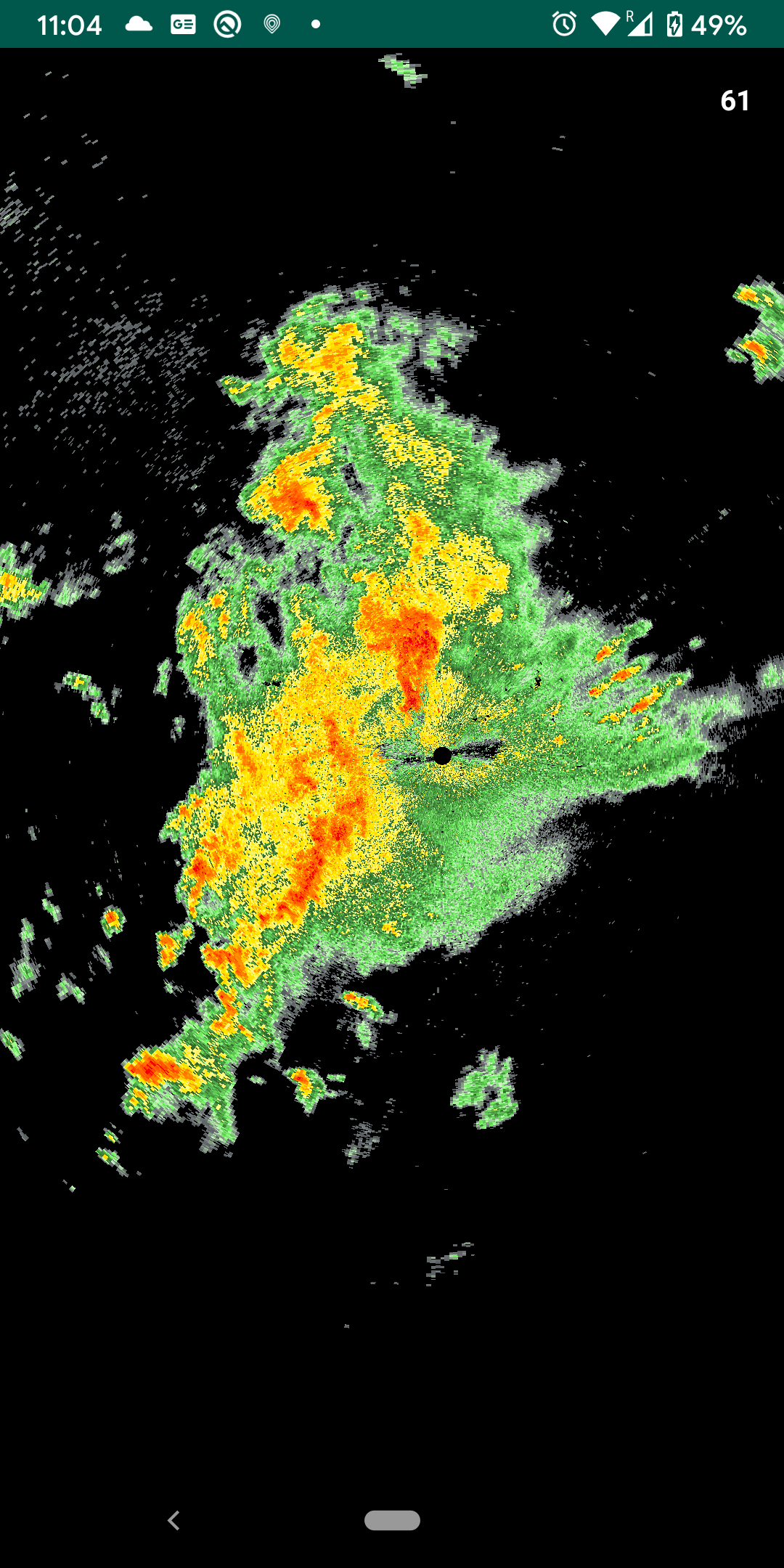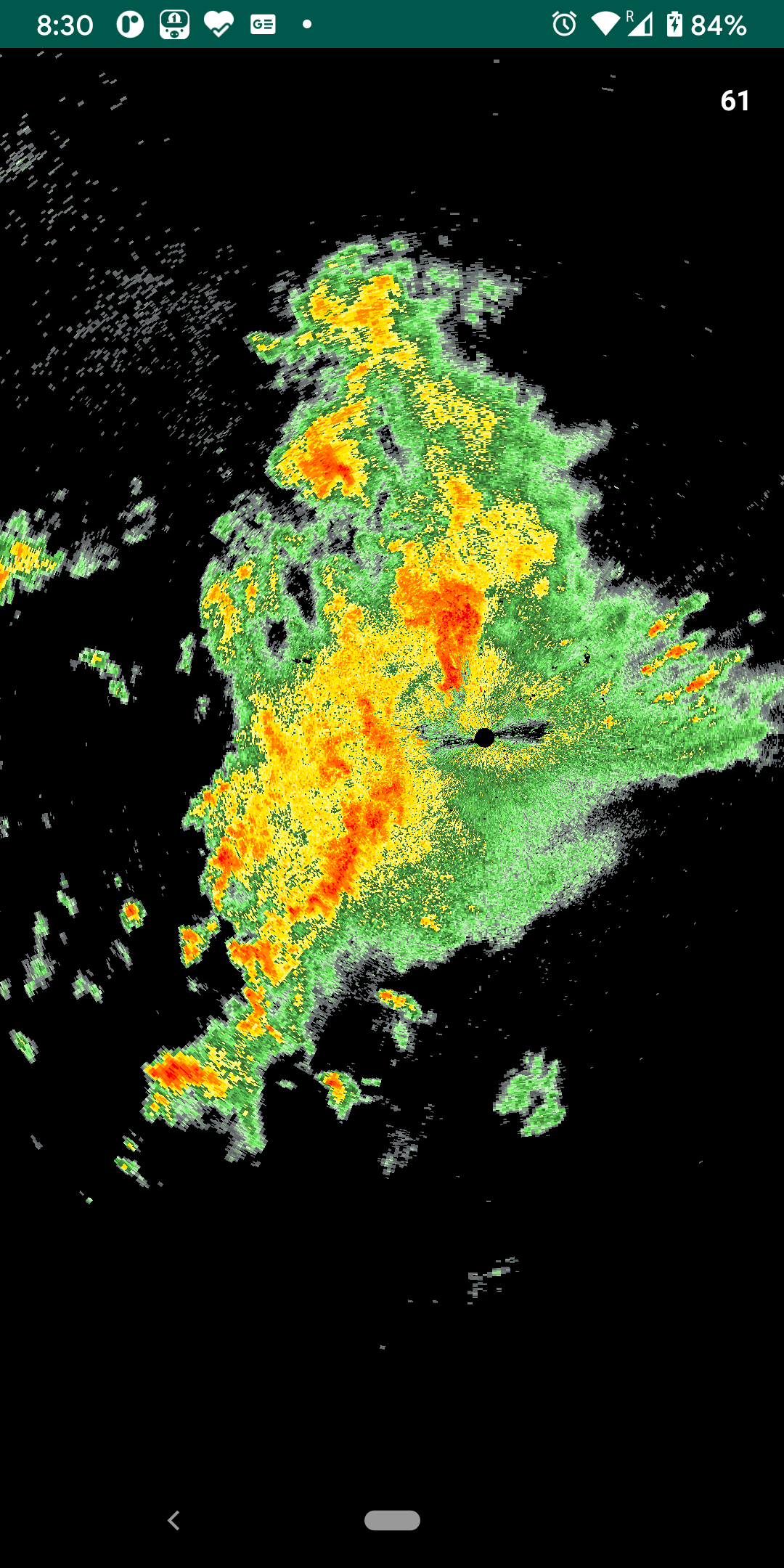Does this look good? We will take a closer look at that, but, here is the complete implementation of Reflectivity Layer with Half data type for now.

## Performance

Performance was the primary reason for this exercise. I found that while data transfer takes half the time preprocessing(generating vertices) is taking 4 times the time. Here are the result of 5 runs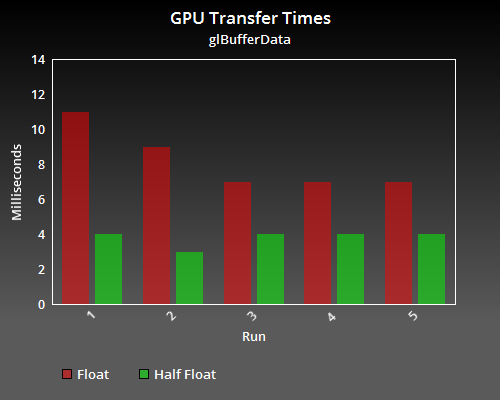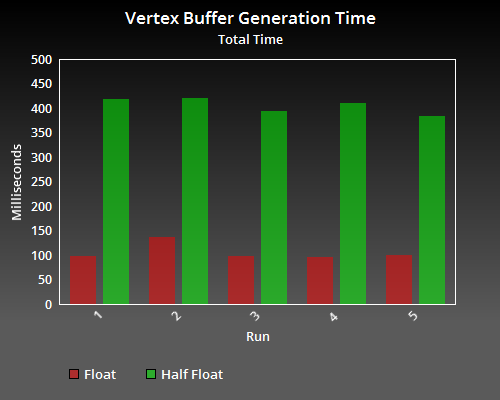Reduced GPU transfer times are because there is only 50% data to transfer as compared to `Float`. Preprocessing time got increased because of extra boxing and un-boxing I believe. In a production app preprocessing will be done off UI thread so this may be okay depending upon the use case. We are still getting 60 FPS which is expected. So far so good, `Half` is looking promising. Let’s have a look at its support.

## Support

Unfortunately, `Half` was introduced in API level 26 and hence can only support Android devices running Android Oreo and above. As most of the Android developers don’t have the luxury of having `minSdk=26`(yet) so one needs to maintain two implementations of the same Layer/Object. That’s exactly what I have done. I created two layers `ReflectivityLayer` and `ReflectivityLayerHalfFloat` and based on the Android version I use the appropriate class.

Also `GL_HALF_FLOAT` requires `OpenGL ES 3.0` and above which most of the devices should be able to support at this point. It’s again up-to-the developer to perform that check and use appropriate implementation.

## Precision

As I have mentioned before 16bit Float comes at the price of precision. A precision table is given in documentation which maps what ranges of values will have what precision. In our case latitude and longitudes vary between [-180, 180]. As per the table storing a value of 128 will give a precision of 1/8 or 0.125. This can introduce error of multiple kilometers when converted. This is evident when zoomed in on half float render. Here is a comparison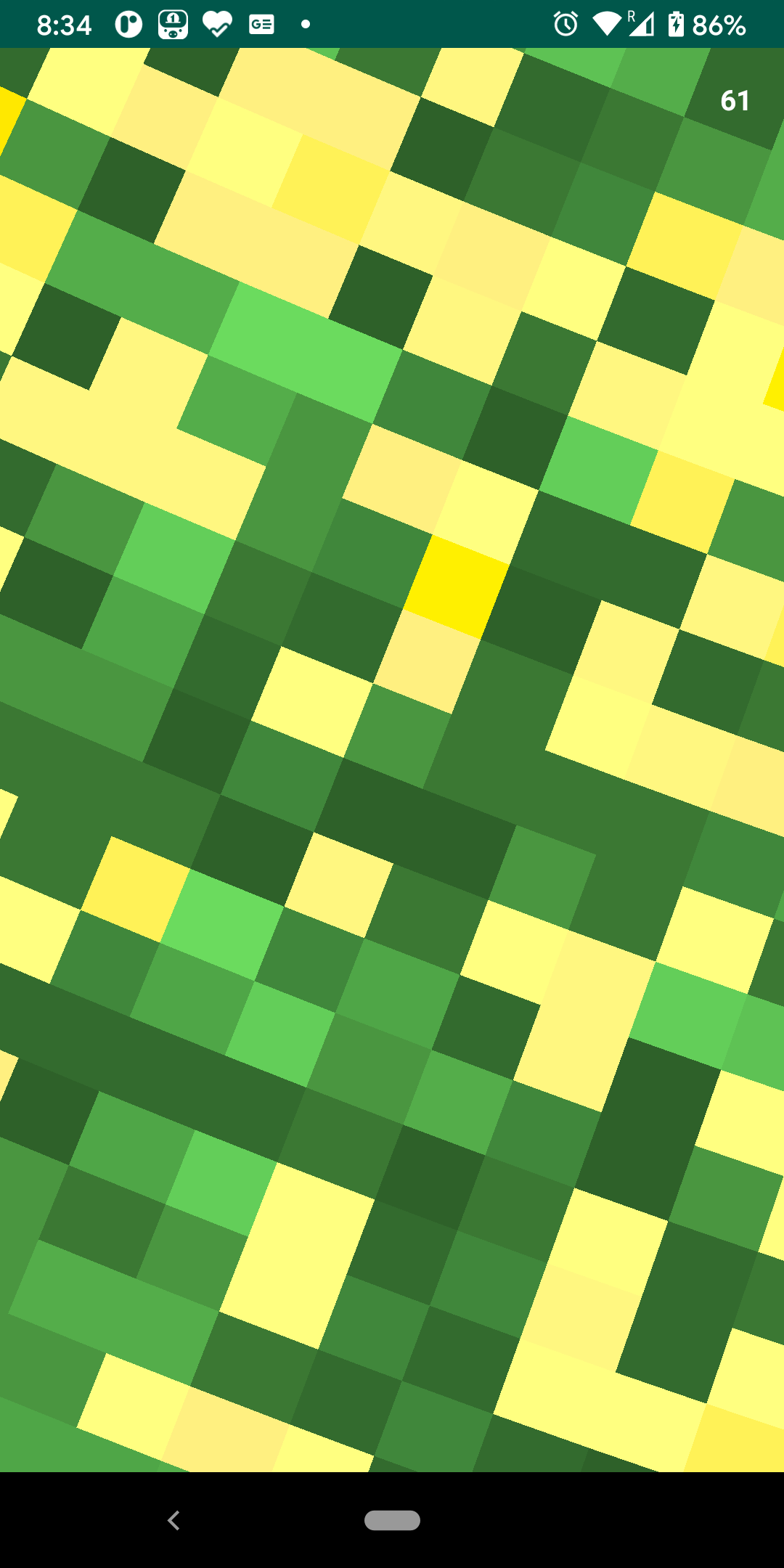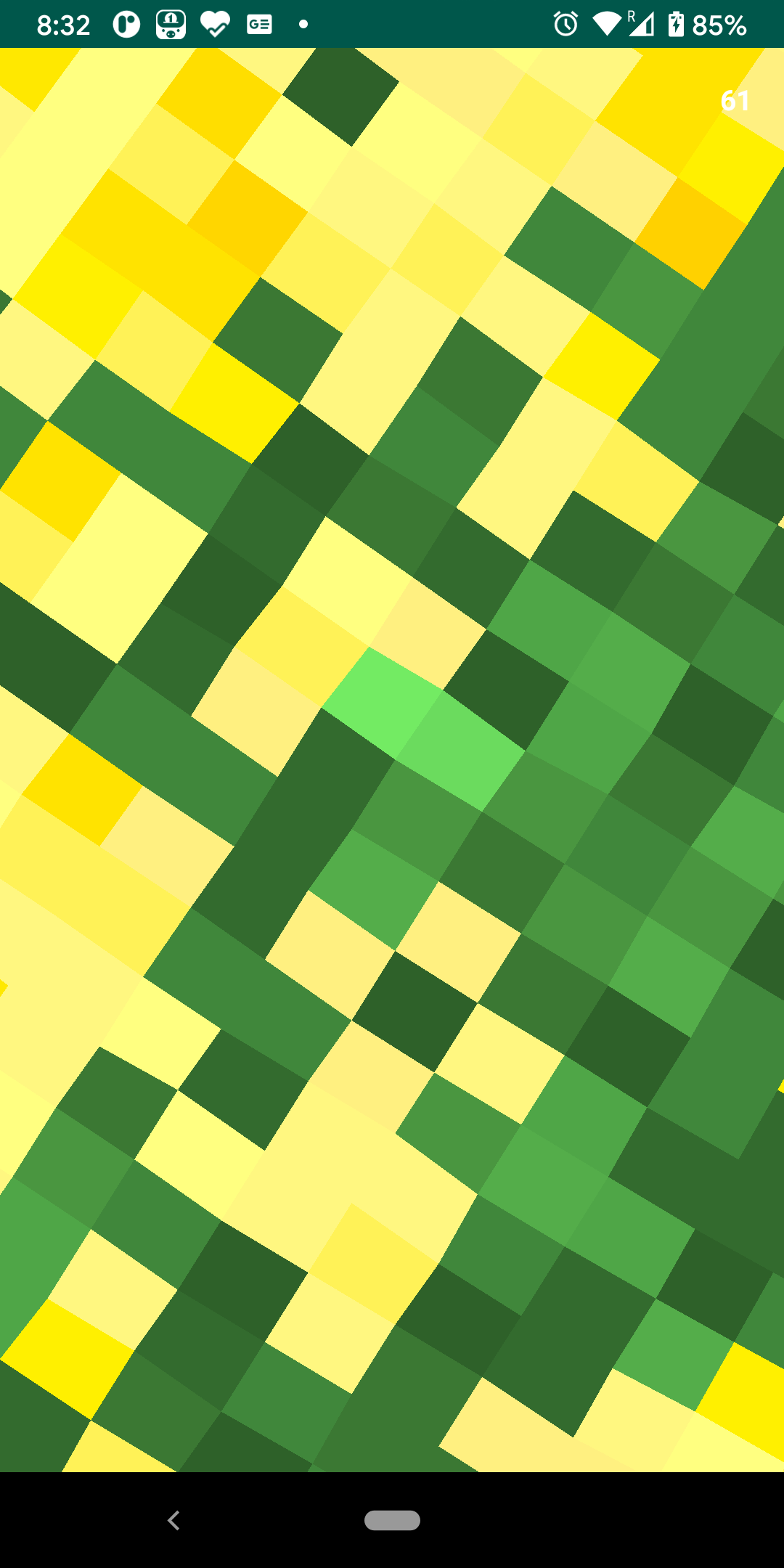The left image represents full 32bit `Float` render, while the right image represents 16bit `Half` render. You can see how lines are zig-zagged in case of half float. This is significant for this use case since some people rely on these renders in realtime.

Because of the above-stated reasons and primarily precision issue, I concluded that this optimization may not be a good idea for my case. In the interest of research and experimentation here is full set of changes.

Happy Coding!